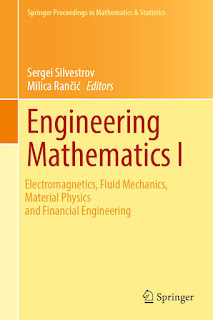ANALYSICS MATHEMATICS

# Engineering Mathematics I Electromagnetics, Fluid Mechanics, Material Physics and Financial Engineering PDF Free DownloadThis book highlights the latest advances in engineering mathematics with a main focus on the mathematical models, structures, concepts, problems and computational methods and algorithms most relevant for applications in modern technologies and engineering. In particular, it features mathematical methods and models of applied analysis, probability theory, differential equations, tensor analysis and computational modelling used in applications to important problems concerning electromagnetics, antenna technologies, fluid dynamics, material and continuum physics and financial engineering. The individual chapters cover both theory and applications, and include a wealth of figures, schemes, algorithms, tables and results of data analysis and simulation. Presenting new methods and results, reviews of cutting-edge research, and open problems for future research, they equip readers to develop new mathematical methods and concepts of their own, and to further compare and analyse the methods and results discussed.